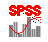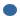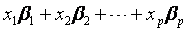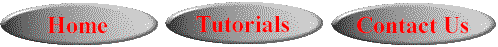# SPSS On-Line Training WorkshopHOME Table of Contents Data Editor Window Syntax  Editor WindowCarl Lee Felix Famoye About Us Chart Editor Window Output Window Overview of Data Analysis Manipulation of Data Analysis of Data Projects & Data Sets Integrate R into SPSS

# Survival AnalysisIn this Tutorial:

Survival Analysis:

Cox Regression

Analysis of DataSurvival Analysis includes Lift Tables, Kaplan-Meier, Cox Regression and Cox Regression with Time Dependent Covariates. The following two movie clips demonstrate how to build  survival models using Kaplan-Meier method and Cox Regression.

In this on-line workshop, you will find many movie clips. Each movie clip will demonstrate some specific usage of SPSS.

Survival Analysis:  Survival time is a common response for studying the effects of treatments on life time. The properties of Survival times are usually characterized by (1) survival function (also known as reliability function, or cumulative survival rate) and (2) hazard function (aslo known as failure rate function). A unique characteristic is that the survival time often is censored. It may be left-censored, right censored or both.  A hazard function of survival time T is the conditional failure rate defined as the probability of failure during a small time interval given the individual has survived. Survival analysis usually studies the survival time based on some treatment effects and the covariates. SPSS provides four techniques for studying survival time.Life Tables: The first step of constructing a lift time table is to subdivide the period of observation into smaller time intervals. For each small time interval, the subjects which are observed at least that long are used to calculate the probability of a terminal event occurring in that interval. The probabilities estimated from each of the intervals are then used to estimate the overall probability of the event occurring at different time points. See SPSS Help Menu for additional information.Kaplan-Meier Model: Kaplan-Meier method is a nonparametric technique for estimating the survival rates with the presence of censored cases. The basic idea is to first compute the conditional probabilities at each time point when an event occurs and then, compute the product limit of those probabilities to estimate the survival rate at each point in time. It is also called Product-Limit method. This technique is often used for comparing the effects of treatments on the survival time.

The following movie clip demonstrates how to build Kaplan-Meier model.

The data set used for this demonstration is Rat Tumor data set. See the Data Set page for details. The data is taken from the study by King et al. (1979). They studied the tumor-free time (in days) of 90 rats on three different diets. The Fat level is the treatment level of three diets: 1 is low fat diet, 2 is saturated fat diet and 3 is unsaturated fat diet. The variable, TumorFreeTime, is the time until the rat developed tumors. Censored is the indicator of uncensored data, coded as 0, and censored data coded as 1.Cox Regression Model: Cox regression model is a common technique used for comparing the survival time among treatment levels and taking into account the covariate effects with the presence of censored cases. This is also known as a proportional hazard model. Proportional hazard model assumes that the covariate effects on a hazard function is the same for different factor levels for all time points. That is, the ratio of the hazard functions for two individuals with values of covariate vectors x1 and x2 does not vary with time t. This implies that the hazard function of T given covariates x can be written as  h(t|x) = h0(t)g(x). h0(t) is the baseline hazard function where g(x) = 1. Cox regression model takes g(x) = exb, where xb =, and p is the number of covariates.

The following movie clip demonstrates how to build Cox regression model.

The data set used for this demonstration is the Lung Cancer data set taken from Prentice (1973), which consists of 40 patients’ survival time due to lung cancer using two different treatments. See the Data Set page for details. The dependent variable is the survival time. The treatment has two levels-1: standard treatment, and 2: experimental treatment. There are also several covariates including medical condition, patient's age, tumor type, and time between diagnosis and treatment.Cox Regression with Time-dependent Covariates: The Cox regression assumes that the covariate effects on a hazard function is the same for different factor levels for all time points. This assumption may not be satisfied. The Cox regression with time-dependent covariates is a technique for modeling survival time with time-dependent covariates. See SPSS Help Menu for additional information.This online SPSS Training Workshop is developed by Dr Carl Lee, Dr Felix Famoye , student assistants Barbara Shelden and Albert Brown , Department of Mathematics, Central Michigan  University. All rights reserved.